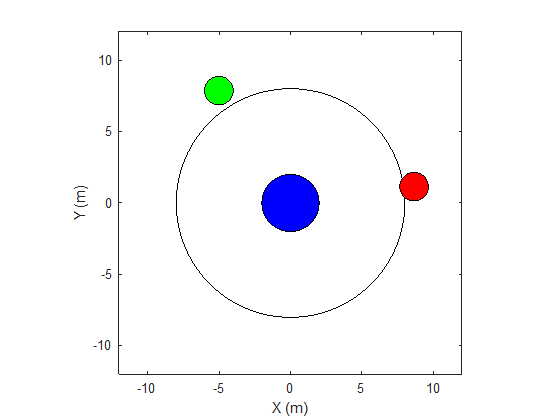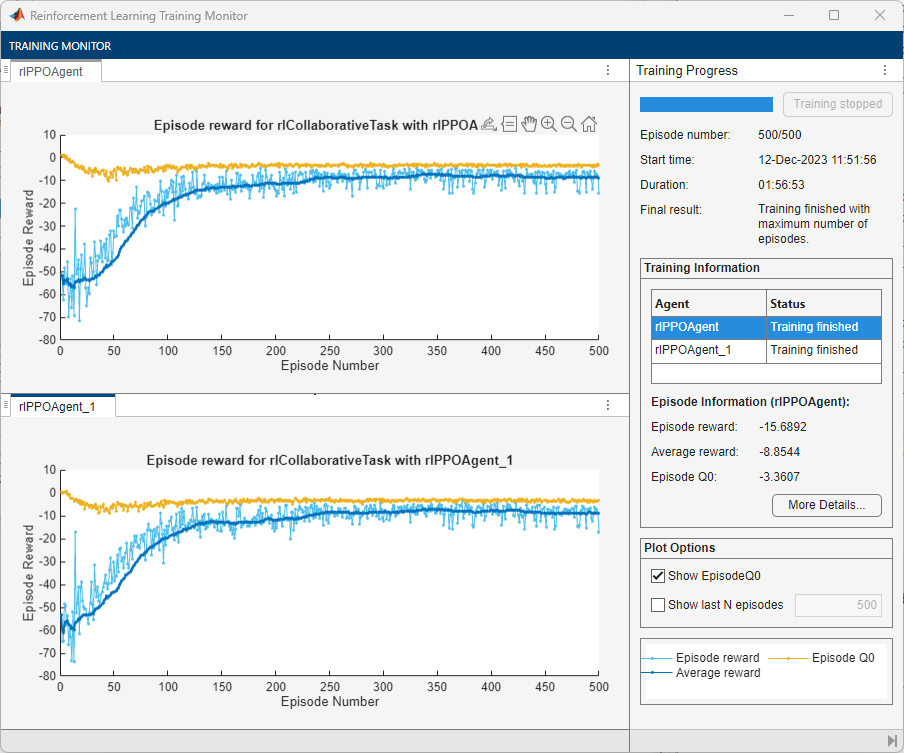# Train Multiple Agents to Perform Collaborative Task

This example shows how to set up a multi-agent training session on a Simulink® environment. In the example, you train two agents to collaboratively perform the task of moving an object.The environment in this example is a frictionless two dimensional surface containing elements represented by circles. A target object C is represented by the blue circle with a radius of 2 m, and robots A (red) and B (green) are represented by smaller circles with radii of 1 m each. The robots attempt to move object C outside a circular ring of a radius 8 m by applying forces through collision. All elements within the environment have mass and obey Newton's laws of motion. In addition, contact forces between the elements and the environment boundaries are modeled as spring and mass damper systems. The elements can move on the surface through the application of externally applied forces in the X and Y directions. There is no motion in the third dimension and the total energy of the system is conserved.

Create the set of parameters required for this example.

`rlCollaborativeTaskParams`

```mdl = "rlCollaborativeTask"; open_system(mdl)```For this environment:

• The 2-dimensional space is bounded from –12 m to 12 m in both the X and Y directions.

• The contact spring stiffness and damping values are 100 N/m and 0.1 N/m/s, respectively.

• The agents share the same observations for positions, velocities of A, B, and C and the action values from the last time step.

• The simulation terminates when object C moves outside the circular ring.

• At each time step, the agents receive the following reward:

`$\begin{array}{l}{\mathit{r}}_{\mathit{A}}={\mathit{r}}_{\mathrm{global}}+{\mathit{r}}_{\mathrm{local},\mathit{A}}\\ {\mathit{r}}_{\mathit{B}}={\mathit{r}}_{\mathrm{global}}+{\mathit{r}}_{\mathrm{local},\mathit{B}}\\ {\mathit{r}}_{\mathrm{global}}=0.001{\mathit{d}}_{\mathit{c}}\\ {\mathit{r}}_{\mathrm{local},\mathit{A}}=-0.005{\mathit{d}}_{\mathrm{AC}}-0.008{\mathit{u}}_{\mathit{A}}^{2}\\ {\mathit{r}}_{\mathrm{local},\mathit{B}}=-0.005{\mathit{d}}_{\mathrm{BC}}-0.008{\mathit{u}}_{\mathit{B}}^{2}\end{array}$`

Here:

• ${\mathit{r}}_{\mathit{A}}$and ${\mathit{r}}_{\mathit{B}}$ are the rewards received by agents A and B, respectively.

• ${\mathit{r}}_{\mathrm{global}}$ is a team reward that is received by both agents as object C moves closer towards the boundary of the ring.

• ${\mathit{r}}_{\mathrm{local},\mathit{A}}$ and ${\mathit{r}}_{\mathrm{local},\mathit{B}}$ are local penalties received by agents A and B based on their distances from object C and the magnitude of the action from the last time step.

• ${\mathit{d}}_{\mathit{C}}$ is the distance of object C from the center of the ring.

• ${\mathit{d}}_{\mathrm{AC}}$ and ${\mathit{d}}_{\mathrm{BC}}$ are the distances between agent A and object C and agent B and object C, respectively.

• ${\mathit{u}}_{\mathit{A}}$ and ${\mathit{u}}_{\mathit{B}}$ are the action values of agents A and B from the last time step.

This example uses proximal policy optimization (PPO) agents with discrete action spaces. To learn more about PPO agents, see Proximal Policy Optimization Agents. The agents apply external forces on the robots that result in motion. At every time step, the agents select the actions ${\mathit{u}}_{\mathit{A},\mathit{B}}=\left[{\mathit{F}}_{\mathit{X}}\text{\hspace{0.17em}},{\mathit{F}}_{\mathit{Y}}\right]$, where ${\mathit{F}}_{\mathit{X}},{\mathit{F}}_{\mathit{Y}}$ is one of the following pairs of externally applied forces.

`${\mathit{F}}_{\mathit{X}}=-1.0\text{\hspace{0.17em}}\mathit{N},\text{\hspace{0.17em}\hspace{0.17em}}{\mathit{F}}_{\mathit{Y}}=-1.0\text{\hspace{0.17em}}\mathit{N}$`

`${\mathit{F}}_{\mathit{X}}=-1.0\text{\hspace{0.17em}}\mathit{N},\text{\hspace{0.17em}\hspace{0.17em}}{\mathit{F}}_{\mathit{Y}}=0$`

`${\mathit{F}}_{\mathit{X}}=-1.0\text{\hspace{0.17em}}\mathit{N},\text{\hspace{0.17em}\hspace{0.17em}}{\mathit{F}}_{\mathit{Y}}=1.0\text{\hspace{0.17em}}\mathit{N}$`

`${\mathit{F}}_{\mathit{X}}=0,\text{\hspace{0.17em}\hspace{0.17em}\hspace{0.17em}\hspace{0.17em}\hspace{0.17em}\hspace{0.17em}\hspace{0.17em}\hspace{0.17em}\hspace{0.17em}\hspace{0.17em}\hspace{0.17em}\hspace{0.17em}\hspace{0.17em}\hspace{0.17em}\hspace{0.17em}}{\mathit{F}}_{\mathit{Y}}=-1.0\text{\hspace{0.17em}}\mathit{N}$`

`${\mathit{F}}_{\mathit{X}}=0,\text{\hspace{0.17em}\hspace{0.17em}\hspace{0.17em}\hspace{0.17em}\hspace{0.17em}\hspace{0.17em}\hspace{0.17em}\hspace{0.17em}\hspace{0.17em}\hspace{0.17em}\hspace{0.17em}\hspace{0.17em}\hspace{0.17em}\hspace{0.17em}\hspace{0.17em}}{\mathit{F}}_{\mathit{Y}}=0$`

`${\mathit{F}}_{\mathit{X}}=0,\text{\hspace{0.17em}\hspace{0.17em}\hspace{0.17em}\hspace{0.17em}\hspace{0.17em}\hspace{0.17em}\hspace{0.17em}\hspace{0.17em}\hspace{0.17em}\hspace{0.17em}\hspace{0.17em}\hspace{0.17em}\hspace{0.17em}\hspace{0.17em}\hspace{0.17em}}{\mathit{F}}_{\mathit{Y}}=1.0\text{\hspace{0.17em}}\mathit{N}$`

`${\mathit{F}}_{\mathit{X}}=1.0\text{\hspace{0.17em}}\mathit{N},\text{\hspace{0.17em}\hspace{0.17em}\hspace{0.17em}\hspace{0.17em}\hspace{0.17em}}{\mathit{F}}_{\mathit{Y}}=-1.0\text{\hspace{0.17em}}\mathit{N}$`

`${\mathit{F}}_{\mathit{X}}=1.0\text{\hspace{0.17em}}\mathit{N},\text{\hspace{0.17em}\hspace{0.17em}}\text{\hspace{0.17em}\hspace{0.17em}\hspace{0.17em}}{\mathit{F}}_{\mathit{Y}}=0$`

`${\mathit{F}}_{\mathit{X}}=1.0\text{\hspace{0.17em}}\mathit{N},\text{\hspace{0.17em}\hspace{0.17em}\hspace{0.17em}\hspace{0.17em}\hspace{0.17em}}{\mathit{F}}_{\mathit{Y}}=1.0\text{\hspace{0.17em}}\mathit{N}$`

### Create Environment

To create a multi-agent environment, specify the block paths of the agents using a string array. Also, specify the observation and action specification objects using cell arrays. The order of the specification objects in the cell array must match the order specified in the block path array. When agents are available in the MATLAB workspace at the time of environment creation, the observation and action specification arrays are optional. For more information on creating multi-agent environments, see `rlSimulinkEnv`.

Create the I/O specifications for the environment. In this example, the agents are homogeneous and have the same I/O specifications.

```% Number of observations numObs = 16; % Number of actions numAct = 2; % Maximum value of externally applied force (N) maxF = 1.0; % I/O specifications for each agent oinfo = rlNumericSpec([numObs,1]); ainfo = rlFiniteSetSpec({ [-maxF -maxF] [-maxF 0 ] [-maxF maxF] [ 0 -maxF] [ 0 0 ] [ 0 maxF] [ maxF -maxF] [ maxF 0 ] [ maxF maxF]}); oinfo.Name = 'observations'; ainfo.Name = 'forces';```

```blks = ["rlCollaborativeTask/Agent A", "rlCollaborativeTask/Agent B"]; obsInfos = {oinfo,oinfo}; actInfos = {ainfo,ainfo}; env = rlSimulinkEnv(mdl,blks,obsInfos,actInfos);```

Specify a reset function for the environment. The reset function `resetRobots` ensures that the robots start from random initial positions at the beginning of each episode.

`env.ResetFcn = @(in) resetRobots(in,RA,RB,RC,boundaryR);`

### Create Agents

PPO agents rely on actor and critic representations to learn the optimal policy. In this example, the agents maintain neural network-based function approximators for the actor and critic.

Create the critic neural network and representation. The output of the critic network is the state value function $\mathit{V}\left(\mathit{s}\right)$ for state $\mathit{s}$.

```% Reset the random seed to improve reproducibility rng(0) % Critic networks criticNetwork = [... featureInputLayer(oinfo.Dimension(1),'Normalization','none','Name','observation') fullyConnectedLayer(128,'Name','CriticFC1','WeightsInitializer','he') reluLayer('Name','CriticRelu1') fullyConnectedLayer(64,'Name','CriticFC2','WeightsInitializer','he') reluLayer('Name','CriticRelu2') fullyConnectedLayer(32,'Name','CriticFC3','WeightsInitializer','he') reluLayer('Name','CriticRelu3') fullyConnectedLayer(1,'Name','CriticOutput')]; % Critic representations criticOpts = rlRepresentationOptions('LearnRate',1e-4); criticA = rlValueRepresentation(criticNetwork,oinfo,'Observation',{'observation'},criticOpts); criticB = rlValueRepresentation(criticNetwork,oinfo,'Observation',{'observation'},criticOpts);```

The outputs of the actor network are the probabilities $\pi \left(\mathit{a}|\mathit{s}\right)$of taking each possible action pair at a certain state $\mathit{s}$. Create the actor neural network and representation.

```% Actor networks actorNetwork = [... featureInputLayer(oinfo.Dimension(1),'Normalization','none','Name','observation') fullyConnectedLayer(128,'Name','ActorFC1','WeightsInitializer','he') reluLayer('Name','ActorRelu1') fullyConnectedLayer(64,'Name','ActorFC2','WeightsInitializer','he') reluLayer('Name','ActorRelu2') fullyConnectedLayer(32,'Name','ActorFC3','WeightsInitializer','he') reluLayer('Name','ActorRelu3') fullyConnectedLayer(numel(ainfo.Elements),'Name','Action') softmaxLayer('Name','SM')]; % Actor representations actorOpts = rlRepresentationOptions('LearnRate',1e-4); actorA = rlStochasticActorRepresentation(actorNetwork,oinfo,ainfo,... 'Observation',{'observation'},actorOpts); actorB = rlStochasticActorRepresentation(actorNetwork,oinfo,ainfo,... 'Observation',{'observation'},actorOpts);```

Create the agents. Both agents use the same options.

```agentOptions = rlPPOAgentOptions(... 'ExperienceHorizon',256,... 'ClipFactor',0.125,... 'EntropyLossWeight',0.001,... 'MiniBatchSize',64,... 'NumEpoch',3,... 'AdvantageEstimateMethod','gae',... 'GAEFactor',0.95,... 'SampleTime',Ts,... 'DiscountFactor',0.9995); agentA = rlPPOAgent(actorA,criticA,agentOptions); agentB = rlPPOAgent(actorB,criticB,agentOptions);```

During training, agents collect experiences until either the experience horizon of 256 steps or the episode termination is reached, and then train from mini-batches of 64 experiences. This example uses an objective function clip factor of 0.125 to improve training stability and a discount factor of 0.9995 to encourage long-term rewards.

### Train Agents

Specify the following training options to train the agents.

• Run the training for at most 1000 episodes, with each episode lasting at most 5000 time steps.

• Stop the training of an agent when its average reward over 100 consecutive episodes is –10 or more.

```maxEpisodes = 1000; maxSteps = 5e3; trainOpts = rlTrainingOptions(... 'MaxEpisodes',maxEpisodes,... 'MaxStepsPerEpisode',maxSteps,... 'ScoreAveragingWindowLength',100,... 'Plots','training-progress',... 'StopTrainingCriteria','AverageReward',... 'StopTrainingValue',-10);```

To train multiple agents, specify an array of agents to the `train` function. The order of agents in the array must match the order of agent block paths specified during environment creation. Doing so ensures that the agent objects are linked to their appropriate I/O interfaces in the environment. Training these agents can take several hours to complete, depending on the available computational power.

The MAT file `rlCollaborativeTaskAgents` contains a set of pretrained agents. You can load the file and to view the performance of the agents. To train the agents yourself, set `doTraining` to `true`.

```doTraining = false; if doTraining stats = train([agentA, agentB],env,trainOpts); else load('rlCollaborativeTaskAgents.mat'); end```

The following figure shows a snapshot of training progress. You can expect different results due to randomness in the training process.### Simulate Agents

Simulate the trained agents within the environment.

```simOptions = rlSimulationOptions('MaxSteps',maxSteps); exp = sim(env,[agentA agentB],simOptions);```For more information on agent simulation, see `rlSimulationOptions` and `sim`.